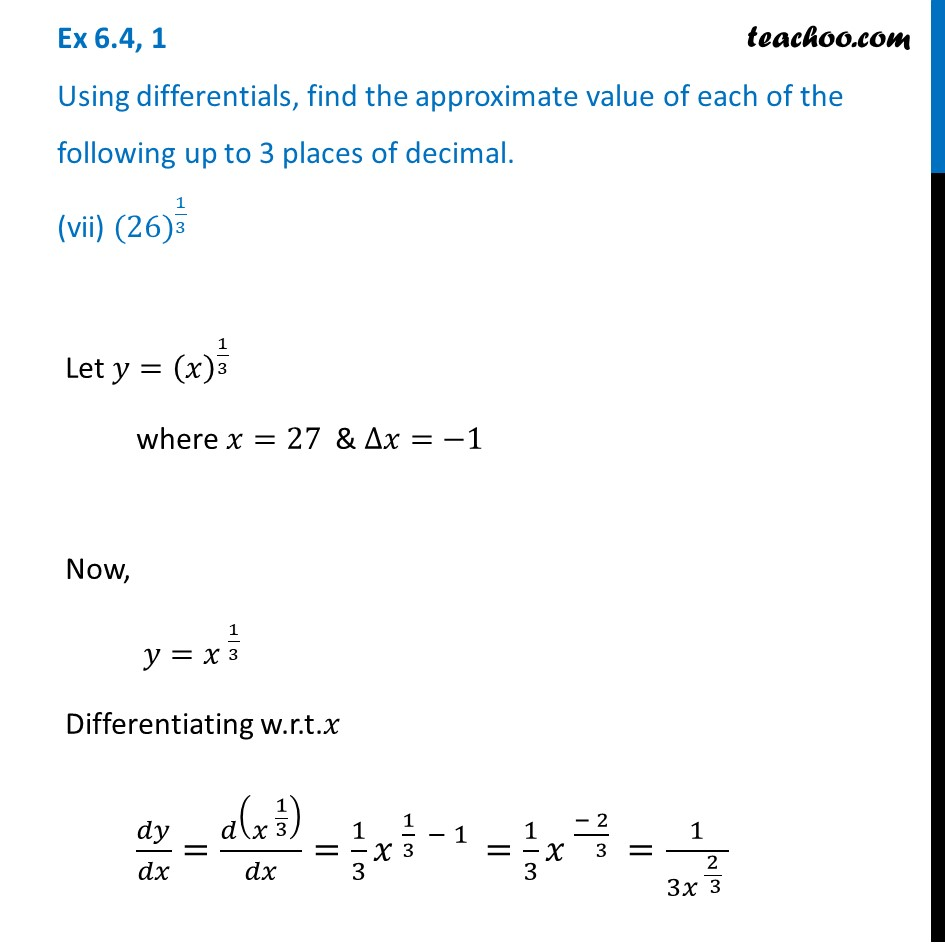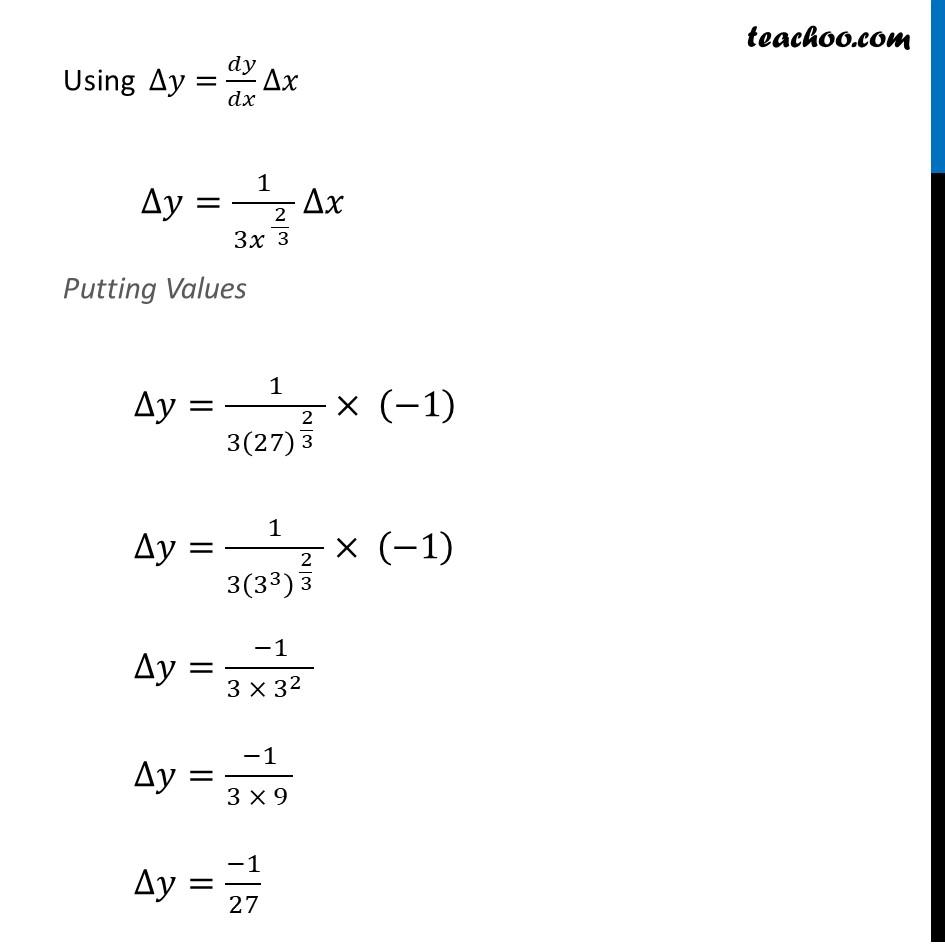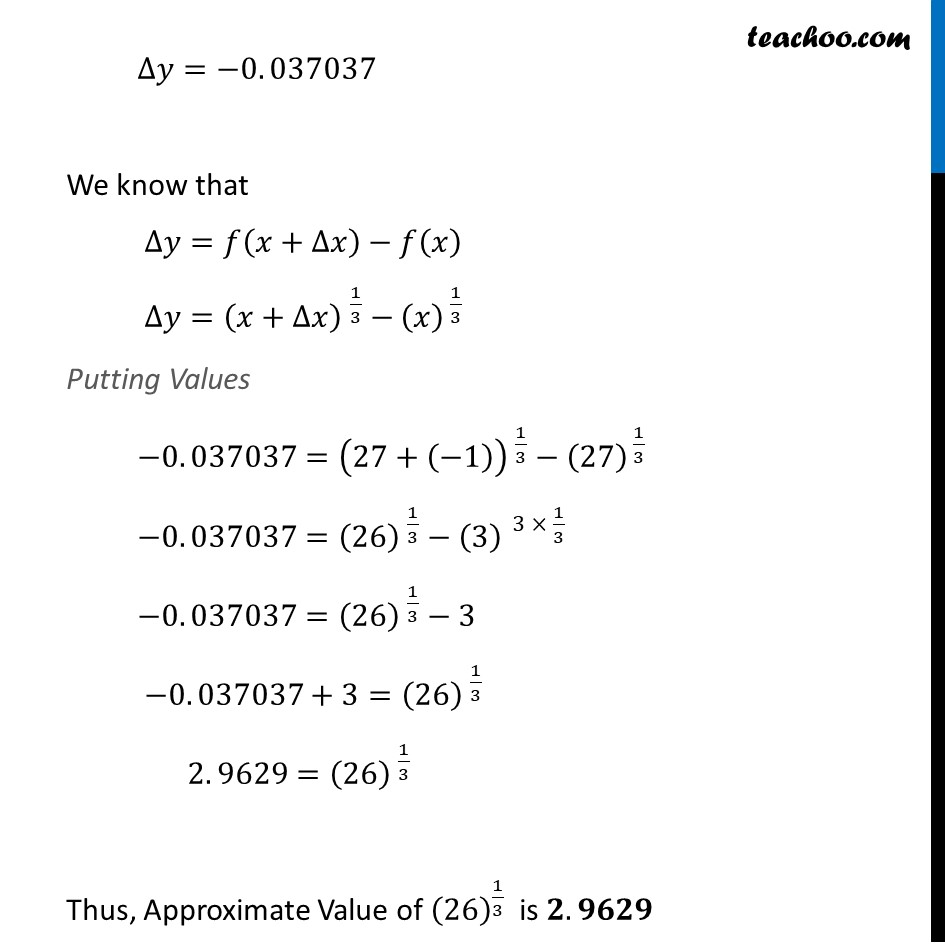Approximations (using Differentiation)

Chapter 6 Class 12 Application of Derivatives
Serial order wiseLearn in your speed, with individual attention - Teachoo Maths 1-on-1 Class

### Transcript

Question 1 Using differentials, find the approximate value of each of the following up to 3 places of decimal. (vii) 〖(26)〗^(1/3)Let 𝑦=(𝑥)^(1/3) where 𝑥=27 & ∆𝑥=−1 Now, 𝑦=〖𝑥 〗^(1/3) Differentiating w.r.t.𝑥 𝑑𝑦/𝑑𝑥=𝑑(〖𝑥 〗^(1/3) )/𝑑𝑥=1/3 〖𝑥 〗^(1/3 − 1) Using ∆𝑦=𝑑𝑦/𝑑𝑥 ∆𝑥 ∆𝑦=1/(3〖𝑥 〗^(2/( 3) ) ) ∆𝑥 Putting Values ∆𝑦=1/(3(27)^( 2/3 ) )× (−1) ∆𝑦=1/(3(3^3 )^( 2/3 ) )× (−1) ∆𝑦=(−1)/(3〖 × 3〗^(2 ) ) ∆𝑦=(−1)/(3 × 9 ) ∆𝑦=(−1)/27 ∆𝑦=−0. 037037 We know that ∆𝑦=𝑓(𝑥+∆𝑥)−𝑓(𝑥) ∆𝑦=〖(𝑥+∆𝑥) 〗^(1/3)−(𝑥)^( 1/3) Putting Values −0. 037037=〖(27+(−1)) 〗^(1/3)−(27)^( 1/3) −0. 037037=〖(26) 〗^(1/3)−(3)^( 3 × 1/3) −0. 037037=〖(26) 〗^(1/3)−3 −0. 037037+3=〖(26) 〗^(1/3) 2. 9629=〖(26) 〗^(1/3) Thus, Approximate Value of (26)^(1/3) is 𝟐. 𝟗𝟔𝟐𝟗 =1/3 〖𝑥 〗^((− 2)/( 3) )=1/(3〖𝑥 〗^(2/( 3) ) ) ∆𝑦=−0. 037037 We know that ∆𝑦=𝑓(𝑥+∆𝑥)−𝑓(𝑥) ∆𝑦=〖(𝑥+∆𝑥) 〗^(1/3)−(𝑥)^( 1/3) Putting Values −0. 037037=〖(27+(−1)) 〗^(1/3)−(27)^( 1/3) −0. 037037=〖(26) 〗^(1/3)−(3)^( 3 × 1/3) −0. 037037=〖(26) 〗^(1/3)−3 −0. 037037+3=〖(26) 〗^(1/3) 2. 9629=〖(26) 〗^(1/3) Thus, Approximate Value of (26)^(1/3) is 𝟐. 𝟗𝟔𝟐𝟗